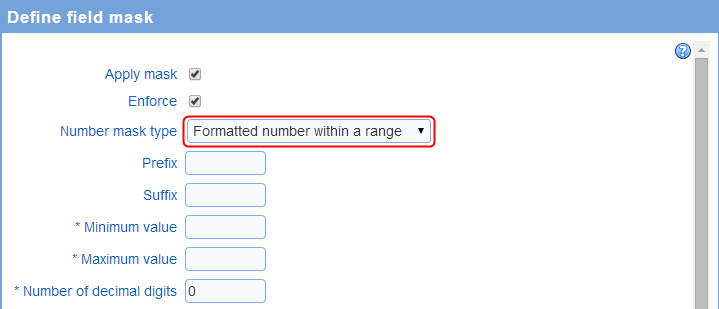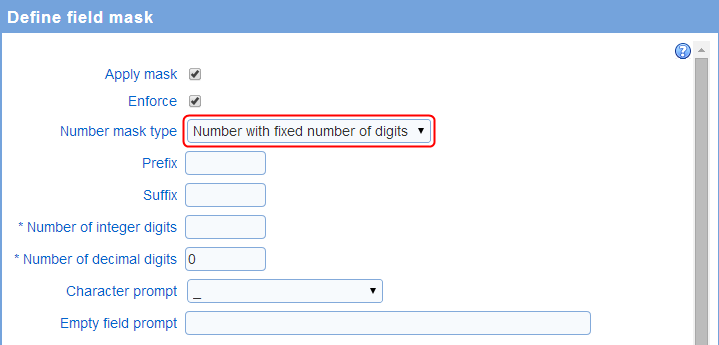The system supports four types of formatted number fields:

• Numbers within a range: allows for numbers within a specific range. Examples of numbers within a range include quantities such as prices, salaries, or age

• Numbers with a fixed number of digits: allows for numbers with a set number of digits. Examples of numbers with a fixed number of digit include postal codes or employee ID

• Auto-formatted numbers: auto-formatted numbers are modified to include separators for groups of digits automatically. For example, number 1000000 will be formatted as 1,000,000 automatically. The formatting is applied when the respondent click or tabs out of the field containing the number. Note that auto-formatting of numbers is supported for cultures that use groups consisting of exactly 3 digits. Other grouping schemes are not supported.

Note that it is possible and often recommended to substitute a masked text field for a number with a fixed number of digits when the value captured should be listed as-is and  does not need to be treated as a number in reporting function (e.g., computing descriptive statistics or plotting the magnitude of the quantity in charts). However, using a masked text field in this situation requires creating a custom mask, which is more complex than using the fields provided for number fields with a fixed number of digits.

Prefix and suffix

The prefix and suffix are text or symbols shown before or after the number in the field, respectively. A common use of a prefix is to include a currency symbol such as \$ or Ą. Examples of suffixes are %, mpg or kWhr.

Formatted numbers within a rangeMinimum value: the minimum number that can be entered in the field

Maximum value: the maximum value that can be entered in the field

Number of decimal digits: the number of decimal digits allowed for the number. If the number of decimal digits is set to 0, then the number entered must be an integer.

Numbers with a fixed number of digitsNumber of integer digits: the number of digits before the decimal point

Number of decimal digits: the number of digits after the decimal point

Character prompt: the character to use to mark the position of each digit for the number

Example: for a number with 5 integer digits and 2 decimal digits and a character prompt of '?', the field will display ?????.?? indicating each of the digits that must be entered.

Empty field prompt: the text to show in the field when it does not have the focus (e.g., the cursor is not in the field). For example, the character prompt may show 'enter your age'.